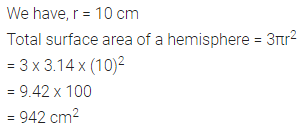# Find the total surface area of a hemisphere of radius 10 cm. (Use π = 3.14)

Find the total surface area of a hemisphere of radius 10 cm. (Use π = 3.14)